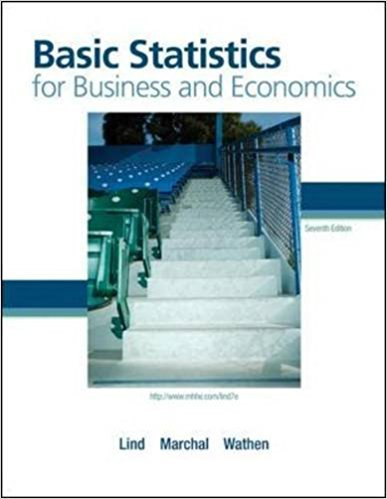×
Get Full Access to Basic Statistics For Business And Economics - 7 Edition - Chapter 6 - Problem 21e
Get Full Access to Basic Statistics For Business And Economics - 7 Edition - Chapter 6 - Problem 21e

×

# In a recent study 90 percent of the homes in the UnitedISBN: 9780077384470 64

## Solution for problem 21E Chapter 6

Basic Statistics for Business and Economics | 7th Edition

• Textbook Solutions
• 2901 Step-by-step solutions solved by professors and subject experts
• Get 24/7 help from StudySoup virtual teaching assistantsBasic Statistics for Business and Economics | 7th Edition

4 5 1 315 Reviews
17
1
Problem 21E

Problem 21E

In a recent study 90 percent of the homes in the United States were found to have large- screen TVs. In a sample of nine homes, what is the probability that:

a. All nine have large-screen TVs?

b. Less than five have large-screen TVs?

c. More than five have large-screen TVs?

d. At least seven homes have large-screen TVs?

Step-by-Step Solution:
Step 1 of 3

Solution 21E

Step1 of 5:

Let us consider a random variable ‘X’ it presents the number of homes in the United States were found to have large- screen TV’s. Also, we have ‘n = 9 and’.

Here our goal is:

a). We need to find the probability that all nine have large-screen TV’s.

b). We need to find the probability that less than five have large-screen TV’s.

c). We need to find the probability that more than five have large-screen TV’s.

d). We need to find the probability at least seven homes have large-screen TV’s.

Step2 of 5:

a).

We know that ‘x’ follows a binomial distribution with parameters ‘n = 9 and’. Then, the probability mass function of the binomial distribution is:Now,Therefore, P(X = 9) = 0.3875.

Step3 of 5:

b).

The probability that less than five have large-screen TV’s is:Where,is obtained from Excel by using the function “=Binomdist(X,n,false)”

 X P(X < 5) 1 8.1E-08 2 2.92E-06 3 6.12E-05 4 0.000827 Total 0.000891

Therefore, P(X < 5) = 0.000891.

Step4 of 5:

c).

The probability that more than five have large-screen TV’s is:Where,Where,is obtained from Excel by using the function “=Binomdist(X,n,false)”

 X P(X5) 1 8.1E-08 2 2.92E-06 3 6.12E-05 4 0.000827 5 0.00744 Total 0.008331

Therefore, P(X5) = 0.008331.

Now,Therefore,Step5 of 5:

d).

The probability at least seven homes have large-screen TV’s is:Where,Where,is obtained from Excel by using the function “=Binomdist(X,n,false)”

 X P(X < 7) 1 8.1E-08 2 2.92E-06 3 6.12E-05 4 0.000827 5 0.00744 6 0.044641 Total 0.0530

Therefore, P(X < 5) = 0.0530.

Now,Therefore,Step 2 of 3

Step 3 of 3

##### ISBN: 9780077384470

Unlock Textbook Solution